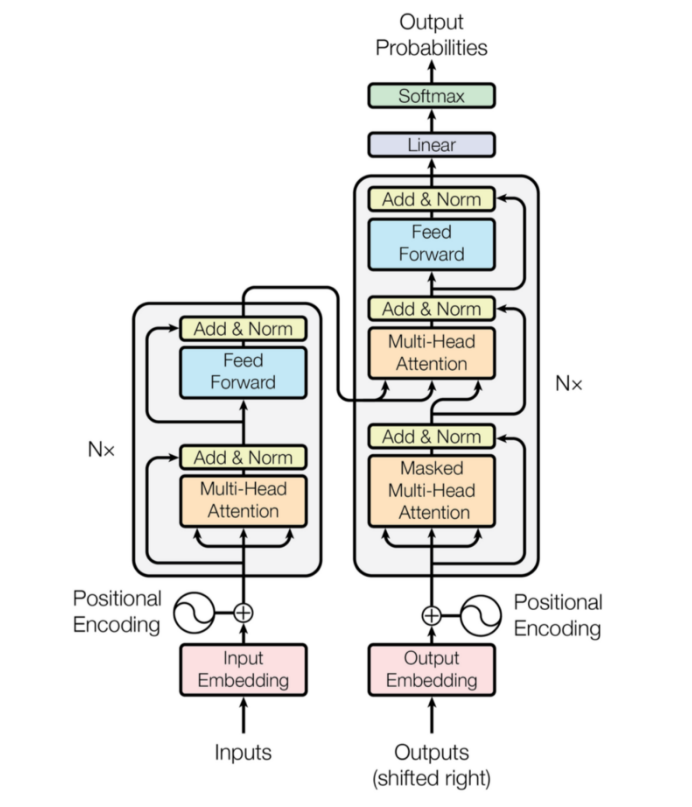# Key Intuition Behind Positional Encodings

## What are Positional Embeddings and where are they used?Transformer Model (Vaswani, et al. 2017)

## Formulation:

• N: Number of word in the sequence
• hʷ: Dimension size of word embedding
• pos: position of the current word in the sequence in [0, N-1]
• i: index of the dimensional index of word embedding in [0, hʷ-1]

## Key Intuition:

`class PositionalEncoding(nn.Module):    def __init__(self, d_model, dropout=0.1, max_len=5000):  super(PositionalEncoding, self).__init__()  self.dropout = nn.Dropout(p=dropout)  pe = torch.zeros(max_len, d_model)  position = torch.arange(0, max_len,dtype=torch.float).unsqueeze(1)  div_term = torch.exp(torch.arange(0, d_model, 2).float()*(-math.log(10000.0) / d_model))          pe[:, 0::2] = torch.sin(position * div_term)          pe[:, 1::2] = torch.cos(position * div_term)          pe = pe.unsqueeze(0).transpose(0, 1)          self.register_buffer('pe', pe)def forward(self, x):  x = x + self.pe[:x.size(0), :]          return self.dropout(x)`